## 内置过渡方式

• `transition_kind` 默认为 0 的内置变量，用于改变切换房间时的过渡效果。注意，该设置是一次性设置，每次切换房间后该变量会自动归零。
• `transition_steps` 设置房间过渡步数的内置变量，默认是 80 步，即 80 步完成过渡过程。

GM8 提供 21 种过渡方式（不计为 0 时的无过渡）：

```1 1 = 从左创造 2 = 从右创造 3 = 从上创造 4 = 从下创造 5 = 中心创造 ```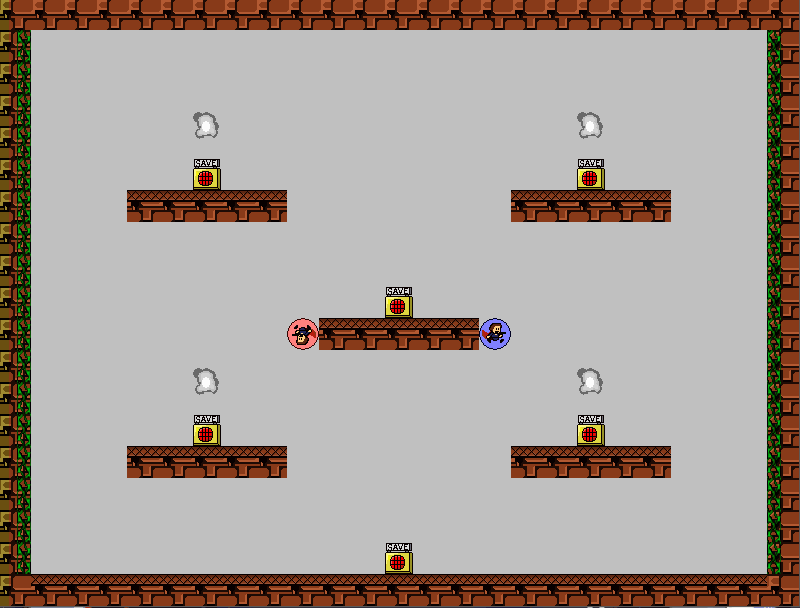```1 6 = 从左移动 7 = 从右移动 8 = 从上移动 9 = 从下移动 ```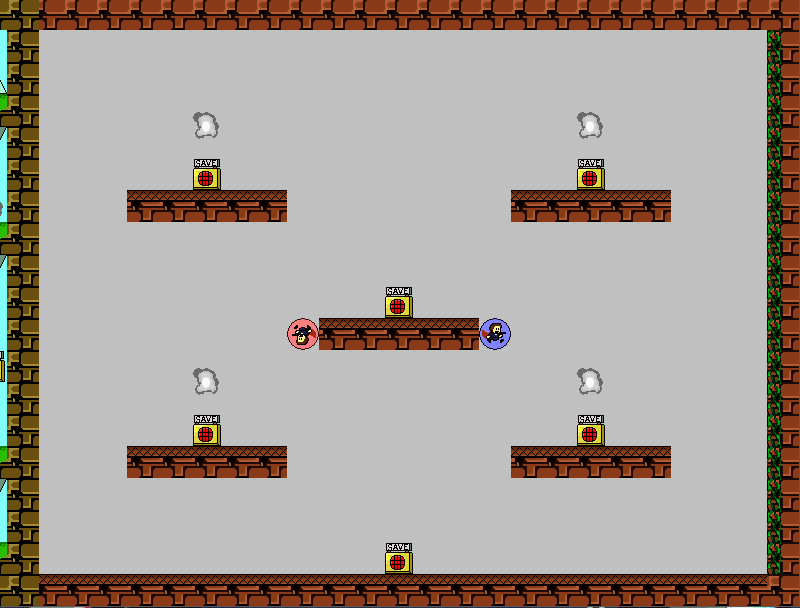```1 10 = 从左隔行 11 = 从右隔行 12 = 从上隔行 13 = 从下隔行 ```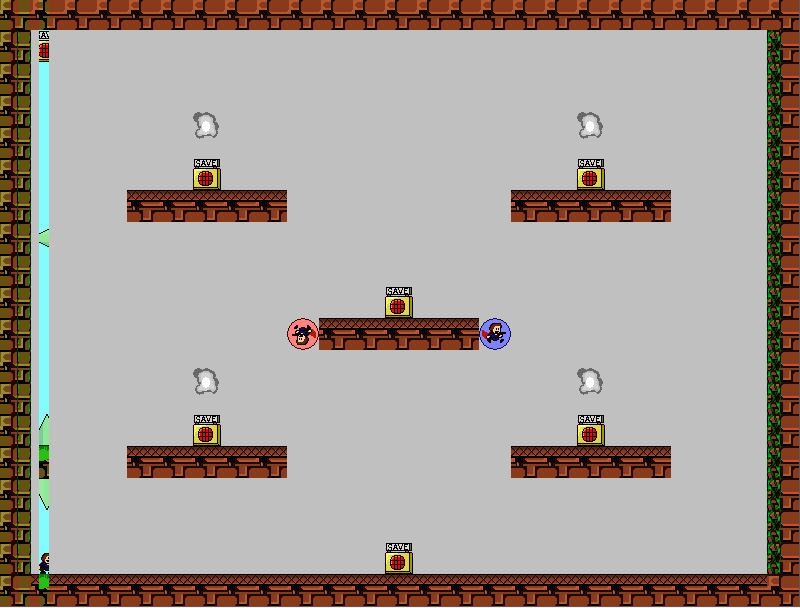```1 14 = 从左推 15 = 从右推 16 = 从上推 17 = 从下推 ``````1 18 = 左转动 19 = 右转动 ```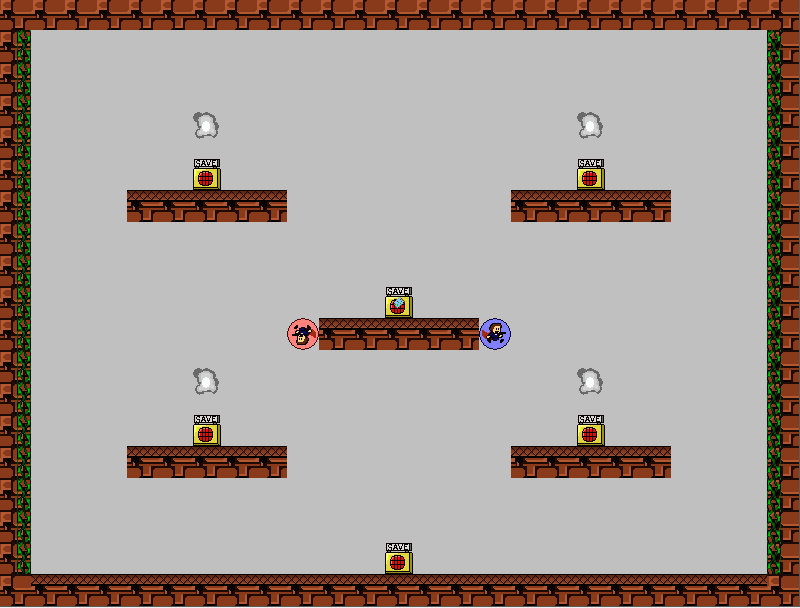```1 20 = 混合房间 ```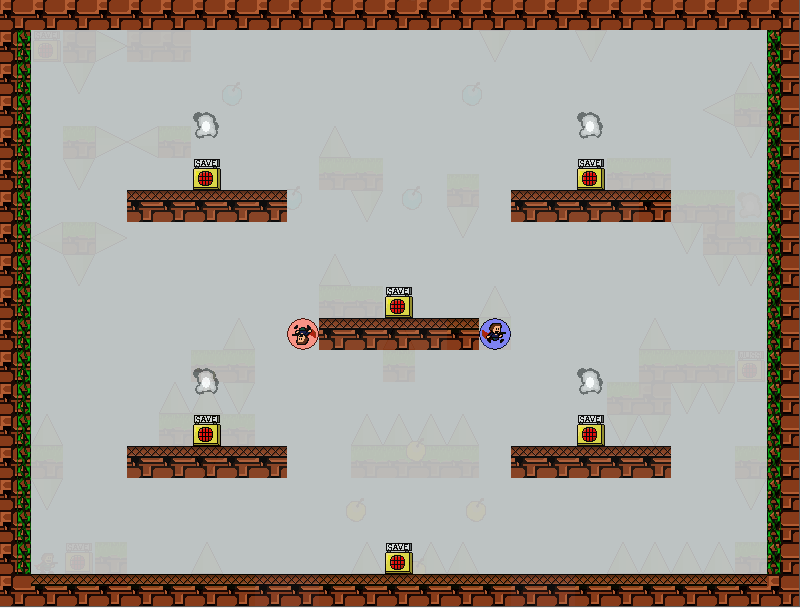```1 21 = 淡入淡出 ```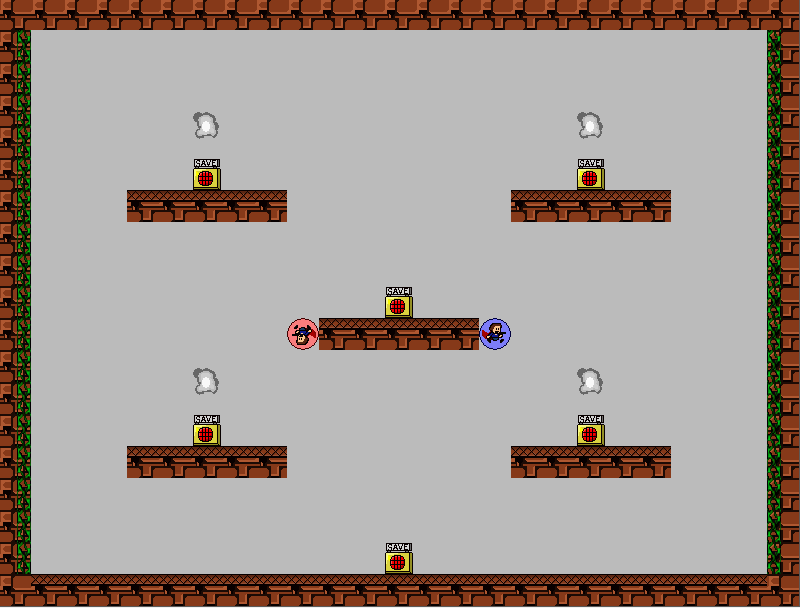## 自定义过渡方式

• `transition_define(kind, name)` 注册一个新的房间过渡方式。参数 kind 是用来提供给 `transition_kind` 的值，重复定义可以覆盖掉原有效果。参数 name 是自定义过渡方式的脚本名，注意脚本名要使用双引号包起，以字符串方式传递。

```1 2 3 4 5 6 7 8 9 10 11 { var _surf1, _surf2, _width, _height, _percent; _surf1 = argument0; _surf2 = argument1; _width = argument2; _height = argument3; _percent = argument4; draw_surface(_surf1, _percent * _width, 0); draw_surface(_surf2, (_percent - 1) * _width, 0); } ```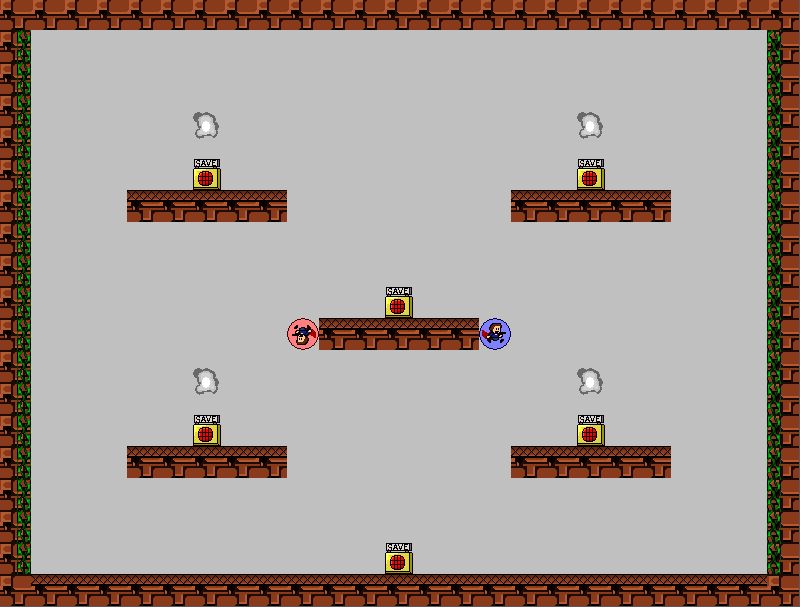```1 2 3 4 5 6 7 8 9 10 11 12 13 { var _surf1, _surf2, _width, _height, _percent; _surf1 = argument0; _surf2 = argument1; _width = argument2; _height = argument3; _percent = argument4; draw_clear(c_black); draw_surface_ext(_surf2, 0, 0, 1, 1, 0, c_white, sqr(_percent)); draw_surface_part(_surf1, 0, 0, _width, _height / 2, 0, -_percent * _height / 2); draw_surface_part(_surf1, 0, _height / 2, _width, _height / 2, 0, (1 + _percent) * _height / 2); } ```